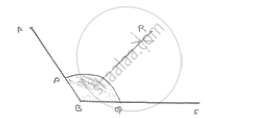# Draw an Obtuse Angle, Bisect It. Measure Each of the Angles So Obtained. - Mathematics

Draw an obtuse angle, Bisect it. Measure each of the angles so obtained.

#### SolutionSteps of construction:
1. Draw angle ABC of^ 120°
2. With center B and any radius, draw an arc which intersects AB at P and BC at Q
3. With center P and Q and radius more than 1/2PQ draw two arcs, with intersect each other
at R.
4. Join BR

∴ ∠ABR = ∠RBC= 60°

Concept: Basic Constructions
Is there an error in this question or solution?

#### APPEARS IN

RD Sharma Mathematics for Class 9
Chapter 16 Constructions
Exercise 16.2 | Q 2 | Page 9

Share